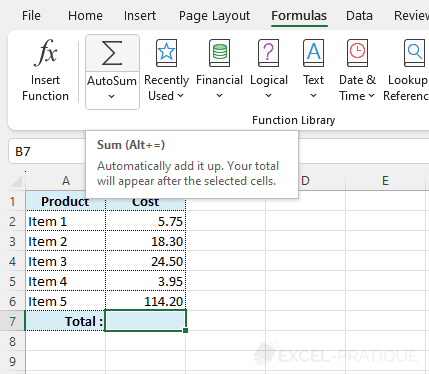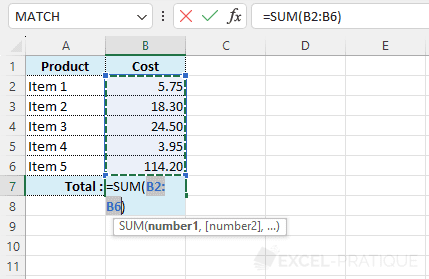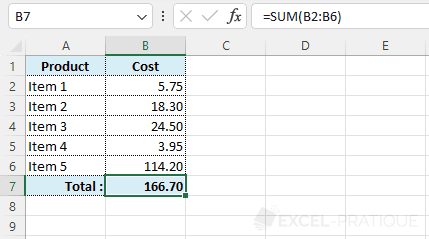# Excel function SUM

As its name suggests, the SUM function calculates the sum of a series of numbers.

Click on the cell where you would like to display the sum and then on the AutoSum button.A list of numbers will be automatically preselected.

If the list is correct, press Enter. If it is not correct, make another selection and then press Enter.The sum will be calculated.